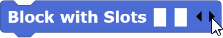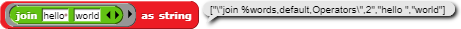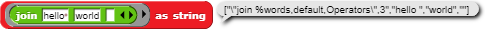# Determine Number of Input Slots?

When a block has been developed that includes the option of multiple input slots, is there a way to detect (via script) how many input slots a user may have created?2 is the number of inputs3 is the number of inputs

The data from the variadic input is returned as a list so you can just find the length of the variable returned

Of course ... that's fantastic. (Thank you!)

Will not work if you put an input list...

It will work because with an input list, it makes each item of the list be an input

I mean:

Input list (for the JOIN block) is considered as 0 inputs.

folks, Glen is asking how to find out inside the block definition how many values a user has entered, and Simon is giving the perfectly straightforward answer: It's the length of the input list. This is - for once - not about your infatuation about syntax analysis